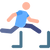top of page# Learning Objectives###### ​###### Jumping to Later Terms of GPsIf we apply the same thinking we used with basic sequences, we can setermine that geometric sequences follow the below format:

a, ar, ar², ar³, ar⁴, ar⁵, where:

• a = the first term; and

• r = the multiplying rate.

This allows us to determine that geometric progressions have an nth term given by the rule:

### Tₙ = a × rⁿ⁻¹

The rule in this format is known as the nth term rule for a GP.###### ExampleThe 15th term of a GP Is 15,667 and the 21st term is 22,224.

1. Find the 18th term.

2. Find the first term.

Solution:

To do this we need to first construct a pair of simultaneous equations using the nth term rule.

15667 = a × r¹⁴

22224 = a × r²⁰

Solve the simultaneous equations to find the value of r.

r = 1.06

Substitute the value of r into one of the equations to find the value of a.

a = 6929.5.

We can now answer the two questions.

1.  T₁₈ = 6929.5 × 1.06¹⁷ = 18659.57.

1. T₁ = 6929.5.###### ​###### ​###### ​###### ​###### ​###### ​bottom of page